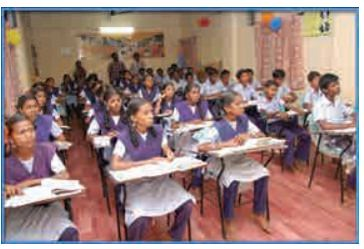Home | | Physics 11th std | Solved Example Problems for Boyle‚Äôs law, Charles‚Äô law and ideal gas law

# Solved Example Problems for Boyle‚Äôs law, Charles‚Äô law and ideal gas law

Thermal Properties Of Matter - Boyle‚Äôs law, Charles‚Äô law and ideal gas law

### EXAMPLE 8.2

A student comes to school by a bicycle whose tire is filled with air at a pressure 240 kPa at 27¬∞C. She travels 8 km to reach the school and the temperature of the bicycle tire increases to 39¬∞C. What is the change in pressure in the tire when the student reaches school?### Solution

We can take air molecules in the tire as an ideal gas. The number of molecules and the volume of tire remain constant. So the air molecules at 27¬∞C satisfies the ideal gas equation P1V1 = NkT1 and at 39¬∞C it satisfies P2V2 = NkT2

But we know### EXAMPLE 8.3

When a person breaths, his lungs can hold up to 5.5 Litre of air at body temperature 37¬∞C and atmospheric pressure (1 atm =101 kPa). This Air contains 21% oxygen. Calculate the number of oxygen molecules in the lungs.### Solution

We can treat the air inside the lungs as an ideal gas. To find the number of molecules, we can use the ideal gas law.

PV = NkT

Here volume is given in the Litre. 1 Litre is volume occupied by a cube of side 10 cm. 1Litre = 10cm √ó 10cm √ó 10cm = 10-3 m3Only 21% of N are oxygen. The total number of oxygen moleculesNumber of oxygen molecules = 2.7 √ó 1022 molecules

### EXAMPLE 8.4

Calculate the volume of one mole of any gas at STP and at room temperature (300K) with the same pressure 1 atm.

### Solution:

Here STP means standard temperature (T=273K or 0¬∞C) and Pressure (P=1 atm or 101.3 kPa)

We can use ideal gas equation V = ŒºRT / P.

By substituting the values=22.4 √ó 10-3 m3

We know that 1 Litre (L) = =10-3m3. So we can conclude that 1 mole of any ideal gas has volume 22.4 L.

By multiplying 22.4L by 300/273KK we get the volume of one mole of gas at room temperature. It is 24.6 L.

### EXAMPLE 8.5

Estimate the mass of air in your class room at NTP. Here NTP implies normal temperature (room temperature) and 1 atmospheric pressure.### Solution

The average size of a class is 6m length, 5 m breadth and 4 m height. The volume of the room V = 6 √ó 5 √ó 4 = 120m3. We can determine the number of mole. At room temperature 300K, the volume of a gas occupied by any gas is equal to 24.6L.

The number of mole Œº=‚âà4878 mol .

Air is the mixture of about 20% oxygen, 79% nitrogen and remaining one percent are argon, hydrogen, helium, and xenon. The molar mass of air is 29 gmol-1.

So the total mass of air in the room = 4878 √ó 29 = 141.4kg.

Study Material, Lecturing Notes, Assignment, Reference, Wiki description explanation, brief detail
11th Physics : UNIT 8 : Heat and Thermodynamics : Solved Example Problems for Boyle‚Äôs law, Charles‚Äô law and ideal gas law |